• HBase 的常用Java API HBase 主要包括 5 大类操作：HBase 的配置、HBase 表的管理、列族的管理、列的管理、数据操作等。 1）org.apache.hadoop.hbase.HBaseConfiguration HBaseConfiguration 类用于管理 HBase 的...
• 本文将介绍String、StringBuilder类的常用方法。 > 在java中**String**类不可变的，创建一个**String**对象后不能更改它的值。所以如果需要对原字符串进行一些改动操作，就需要用**StringBuilder**类或者**...


文章目录
摘要String:String的遍历String的比较搜索子字符串字符串拆分提取子字符串子串的替换转换大小写将其他类型的数据转化为字符串
StringBulider构造方法遍历增加删除修改查找字符串反转字符串截取

摘要

本文将介绍String、StringBuilder类的常用方法。 在java中String类不可变的，创建一个String对象后不能更改它的值。所以如果需要对原字符串进行一些改动操作，就需要用StringBuilder类或者StringBuffer类，StringBuilder比StringBuffer更快一些，缺点是StringBuilder不是线程安全的，但在算法竞赛中一般我们用不到多线程。所以，主要推荐使用StringBuilder类。

了解其他JAVA 常用API和算法点这里 >> https://blog.csdn.net/GD_ONE/article/details/104061907

String:

方法概述： String 类包括的方法可用于检查序列的单个字符、比较字符串、搜索字符串、提取子字符串、创建字符串副本并将所有字符全部转换为大写或小写。

String的遍历

String有两种遍历方式，第一种charAt()方法 第二种是先转化为字符数组，再挨个遍历 charAt(int i);//返回索引i处的字符 length();//返回此字符串的长度 isEmpty();//判空 当length()为0时返回true

String s = "123456";
for(int i = 0; i < s.length(); i++)
System.out.println(s.charAt(i)+" ");// 1 2 3 4 5 6


输出：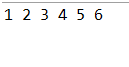toCharArray();//返回此字符串的字符数组

String s = "123456";
char[] s1 = new char;
s1 = s.toCharArray();
for(int i = 0; i < s1.length; i++){
System.out.print(s1[i]+" ");// 1 2 3 4 5 6

输出：String的比较

compareTo(String anotherString)//按字典顺序比较两个字符串 compareToIgnoreCase(String anotherString)//按字典顺序且不区分大小写比较两个字符串 equals(String anotherString)//判断两个字符串是否相等，相等返回true否则返回false equalsIgnoreCase(String str)//同上，不区分大小写。

String s = "abcde";
String s1 = "Abcde";

int f = s.compareTo(s1);
int f1 = s1.compareToIgnoreCase(s);
Boolean f2 = s.equals(s1);
Boolean f3 = s.equalsIgnoreCase(s1);

System.out.println(f);// 32
System.out.println(f1); // 0
System.out.println(f2); // false
System.out.println(f3); // true

输出：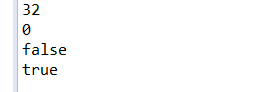compareTo()和compareToIgnoreCase()方法的返回值： a.compareTo(b) 如果a > b 返回大于0的整数 如果a == b 返回0 如果a < b 返回小于0的整数 其实是返回a和b第一个不同字符的差值。

搜索子字符串

indexOf(int ch);// 返回指定字符在此字符串中第一次出现的索引 indexOf(int ch, int fromindex); // 同上， 从指定索引开始搜索 indexOf(String str);//返回子串在此字符串中第一次出现的索引 indexOf(String str, int fromindex);同上，从指定索引开始搜索

lastIndexOf(int ch);//返回指定字符在此字符串最后一次出现的索引 lastIndexOf(int ch, int fromindex);//同上， 从指定索引开始搜索 lastIndexOf(String str);//返回子串在此字符串最后一次出现的索引 lastIndexOf(String str, int fromindex);//同上， 从指定索引开始搜索

startsWith(String prefix);// 检查是否以某一前缀开始

(以上如果不存在，均返回 -1,如果要判断某个字符，应传入字符的Unicode编码)
String s = "12345346";
String s1 = "34";
int f = s.indexOf(s1); // 从索引0开始搜索
int f1 = s.indexOf(s1, 6); // 从索引6开始搜索
int f2 = s.lastIndexOf(s1);
Boolean f3 = s.startsWith("12");
System.out.println(f); // 2
System.out.println(f1);// -1
System.out.println(f2);// 5
System.out.println(f3);// true

输出：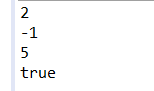字符串拆分

split(String regex); // 根据正则表达式拆分

String s = "ABC DEF";
String s1[] = s.split(" ");//根据空格拆分
System.out.println(s1);// ABC
System.out.println(s1);// DEF

输出：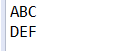提取子字符串

substring(int beginIndex, int endIndex)；//返回从begin开始到end-1结束的子串

String s = "123456";
String s1 = s.substring(0,3);// 123
System.out.println(s1);

输出：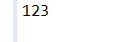子串的替换

replaceAll(String s1,String s2);//用s2替换目标字符串中出现的所有s1 replaceFirst(String s1,String s2);//用s2替换目标字符串中出现的第一个s1

String s = "11123456";
String s1 = s.replaceAll("1", "a");
String s2 = s.replaceFirst("1","a");
System.out.println(s1);///aaa23456
System.out.println(s2);///a1123456


输出：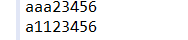转换大小写

toUpperCase(); //将此字符串中的所有字母都换为大写 toLowerCase()//将此字符串中的所有字母都换为小写

将其他类型的数据转化为字符串

valueOf(char[] data);//返回 char数组的字符串表示形式 valueOf(char[] data,int offset, int count)//返回 char 数组参数的特定子数组的字符串表示形式。 valueOf(int i);//返回 int 参数的字符串表示形式。

int a = 10;
String s = String.valueOf(a);
System.out.print(s); // 10

输出： 10
StringBulider
一个可变的字符序列。
构造方法

StringBuilder();//构建一个空的可变字符串。 StringBuilder(String str);//构建一个值为str的可变字符串。

StringBuilder s = new StringBuilder();//
StringBuilder s1 = new StringBuilder("123456");//123456

遍历

charAt(int i);// 返回索引i位置的字符 length();//返回此字符串的长度

StringBuilder s = new StringBuilder("123");
for(int i = 0; i < s.length(); i++)
System.out.println(s.charAt(i)+" ");// 1 2 3

输出：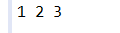增加

append(String str);//在此字符串追加str。 append(StringBuilder str);//在此字符串追加str。 append(char[] str, int offset, int len);//将char的子数组追加到此字符串

StringBuilder s = new StringBuilder("123");
StringBuilder s1 = new StringBuilder("456");
s.append(s1);
System.out.print(s);// 123456

输出：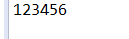删除

delete(int start, int end);//移除此序列从start到end-1的字符串 deleteCharAt(int index);//移除指定索引上的char

StringBuilder s = new StringBuilder("123");
StringBuilder s1 = new StringBuilder("456");
s.delete(0, 1);
s1.deleteCharAt(1);
System.out.println(s);// 23
System.out.println(s1);// 46

输出：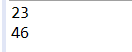修改

setCharAt(int index, char ch);//将指定索引处的字符替换为ch

查找

indexOf(String str);//返回子字符串第一次出现的索引 indexOf(String str, int fromIndex);//同上，从指定位置查找
lastIndexOf(String str);//返回子字符串最后一次出现的索引 lastIndexOf(String str, int fromIndex);//同上，从指定位置查找

字符串反转

reverse();//将此字符串反转

字符串截取

substring(int start);//返回此字符串从start开始至length-1结束的String substring(int start, int end);//返回此字符串从start开始至end-1结束的String toString();//返回此序列中的String表示形式。 (注意以上方法的返回值都是String而不是StringBuilder)

展开全文蓝桥杯 字符串
• ## 算法竞赛中的常用JAVAAPI ：Math类

千次阅读 多人点赞 2020-01-18 21:45:53
Math类包含了一些常用的数学方法,在java....(不能用于大数类，大数类请参见备战蓝桥杯java（五）：算法竞赛中的常用API ：大数类) 求最值 求平方根 求绝对值 求幂（a^b） 取整 得到一个随机数 三角函数 求最值 最小值

Math类包含了一些常用的数学方法,在java.lang.*包内。 (不能用于大数类，大数类请参见备战蓝桥杯java（五）：算法竞赛中的常用API ：大数类)

了解其他JAVA 常用API和算法点这里 >> https://blog.csdn.net/GD_ONE/article/details/104061907

文章目录
求最值求平方根求绝对值求幂（a^b）取整得到一个随机数三角函数

求最值
最小值

Math.min(int a, int b) Math.min(float a, float b) Math.min(double a, doubleb) Math.min(long a, long b)

最大值

Math.max(int a, int b) Math.max(float a, float b) Math.max(double a, doubleb) Math.max(long a, long b)

Math.min() 和 Math.max() 方法分别返回一个最小值和一个最大值。 实例：
public class Main{
public static void main(String[] args){
int a = 10;
int b = 20;
System.out.println(Math.min(a, b));
System.out.println(Math.max(a, b));
}
}


求平方根

Math.sqrt(double a) 返回正确舍入的 double 值的正平方根。

求绝对值

Math.abs(double a) Math.abs(int a) Math.abs(flota) Math.abs(long) 返回一个类型和参数类型一致的绝对值

public class Main{
public static void main(String[] args){
int a = -10;
System.out.println(Math.abs(a));
}
}

求幂（a^b）

Math.pow(double a, double b) 注意无论是传入int还是long都会返回一个double类型的数。

实例：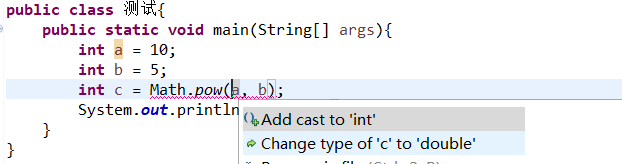所以要求int类型的幂时，要对结果进行类型转换。
取整

Math.ceil(double x) 向上取整，返回大于该值的最近 double 值
System.out.println(Math.ceil(1.4)); // 2.0
System.out.println(Math.ceil(-1.6)); // -1.0


Math.floor(double x) 向下取整，返回小于该值的最近 double 值
System.out.println(Math.floor(1.6)); // 1.0
System.out.println(Math.floor(-1.6)); // -2.0


Math.round(double x); 四舍五入取整
System.out.println(Math.round(1.1)); // 1
System.out.println(Math.round(1.6)); // 2
System.out.println(Math.round(-1.1)); // -1
System.out.println(Math.round(-1.6)); // -2

得到一个随机数

Math.random() 生成一个[0,1)之间的double类型的伪随机数

所以为了得到一个[1, b] 之间的整数可以这样做：
int a = (int)(Math.random()*b + 1); // [1, b]

如果要得到[a, b]的一个整数则是：
int a = (int)(Math.random()*(b - a + 1) + a)  // + 1 是因为random()最大取不到1,所以上限取整后就会少1.

三角函数

Math.cos(double a) 余弦Math.acos(double a) 反余弦Math.sin(double a) 正弦值Math.asin(double a) 反正弦值Math.tan(double a) 正切值Math.atan(double a) 反正切

我们可以用acos()方法求π 因为 cos(π) = -1 所以 acos(-1) = π
展开全文蓝桥杯 数学 Math
• ## 算法竞赛中的常用JAVAAPI ：大数类

千次阅读 多人点赞 2020-01-14 13:40:23
java中的基础数据类型能存储的最大的二进制数是2^63-1，对应的十进制数是9223372036854775807，也就是说只要运算过程中会超过这个数，就会造成数据溢出， BigInteger BigInteger是java.math.* ...


大数类
摘要BigInteger 和 BigDecimal创建赋值加法减法乘法除法*取余*求最大公因数求最值*(a^b)%mod比较大小*进制转化类型转化-BigDecimal的精度问题保留n位小数

摘要

java中的基础数据类型能存储的最大的二进制数是 2 ^ 63 - 1, 对应的十进制数是9223372036854775807，也就是说只要运算过程中会超过这个数，就会造成数据溢出，从而造成错误.
而java.math.*包中提供了大数类，其理论上可以存储无限位的大数，只要内存足够的话。大数类又分为整数和浮点数.即BigInteger and BigDecimal大数类的对象不能直接进行运算，需要调用类中相应的方法,并且方法的参数必须和调用的类相同,BigInteger不能调用BigDecimal, 不能作为其方法参数, 即整数和浮点数不能混合运算.本文举例了一些常用的方法，不需要背会，需要用的时候查java API就行了。

了解其他常用API和算法点这里 >> https://blog.csdn.net/GD_ONE/article/details/104061907
BigInteger 和 BigDecimal
创建
//1.直接声明
BigInteger a;
BigDecimal b;
//2.使用构造函数初始化
BigInteger a = new BigInteger("123456789101112131415");
BigDecimal b = new BigDecimal("123456.123456");

赋值

BigInteger.valueOf(long val); BigDecimal.valueOf(double val);
BigInteger a;
BigDecimal b;
//注意 val 不能超过 long 类型的最大取值9223372036854775807, 超过int时要在数后面加L如:
a = BigInteger.valueOf(123456789101112L); //大于int范围的要加L
b = BigDecimal.valueOf(123456.12341235); // 超出的小数位数会自动舍弃


使用 = 将同类型变量的值赋给另一个变量
BigInteger a;
BigInteger b = new BigInteger("123456");
a = b;
System.out.print(a);

输出:
123456

加法

BigInteger a, b, c;
a = BigInteger.valueOf(123456789); // 赋值为 123456789
b = BigInteger.valueOf(987654321); // 赋值为 987654321
System.out.print(c);
输出：
1111111110
1111111110


减法

BigInteger.subtract(BigInteger); BigDecimal.sbutract(BigDecimal);

BigInteger a, b, c;
a = BigInteger.valueOf(123456789); // 赋值为 123456789
b = BigInteger.valueOf(987654321); // 赋值为 987654321
c = a.subtract(b);
System.out.print(c);

输出：
-864197532


乘法

BigInteger.multiply(BigInteger); BigDecimal.multiply(BigDecimal);

BigInteger a, b, c;
a = BigInteger.valueOf(123456789); // 赋值为 123456789
b = BigInteger.valueOf(987654321); // 赋值为 987654321
c = a.multiply(b);
System.out.print(c);

输出：
121932631112635269


除法

BigInteger.divide(BigInteger); BigDecimal.divide(BigDecimal);

BigInteger a, b, c;
a = BigInteger.valueOf(987654321); // 赋值为 987654321
b = BigInteger.valueOf(123456789); // 赋值为 123456789
c = a.divide(b); // 整数相除仍为整数
System.out.print(c);

输出：
8


*取余

BigInteger.mod(BigInteger);

BigInteger a, b, c;
a = BigInteger.valueOf(987654321); // 赋值为 987654321
b = BigInteger.valueOf(123456789); // 赋值为 123456789
c = a.mod(b);
System.out.print(c);

输出：
9

*求最大公因数

BigInteger.gcd(BigInteger);

BigInteger a, b, c;
a = BigInteger.valueOf(987654321); // 赋值为 987654321
b = BigInteger.valueOf(123456789); // 赋值为 123456789
c = a.gcd(b);
System.out.print(c);

输出：
9


求最值

BigInteger.max(BigInteger) , BigDecimal.max(BigDecimal) 最大值 BigInteger.min(BigInteger) , BigDecimal.min(BigDecimal) 最小值

BigInteger a, b, c, d;
a = BigInteger.valueOf(987654321); // 赋值为 987654321
b = BigInteger.valueOf(123456789); // 赋值为 123456789
c = a.max(b); //a,b中的最大值
d = a.min(b); //a,b中的最小值
System.out.println(c);
System.out.println(d);

输出：
987654321
123456789


*(a^b)%mod

BigInteger.modPow(BigInteger, BigInteger);

BigInteger a, b, c, mod;
a = BigInteger.valueOf(987654321); // 赋值为 987654321
b = BigInteger.valueOf(123456789); // 赋值为 123456789
mod = BigInteger.valueOf(10007);
c = a.modPow(b, mod); //(a^b)%mod
System.out.println(c);
输出：
718


比较大小

BigInteger.compareTo(BigInteger); BigDecimal.compareTo(BigDecimal);

BigInteger a, b;
int c;
a = BigInteger.valueOf(987654321); // 赋值为 987654321
b = BigInteger.valueOf(123456789); // 赋值为 123456789
c = a.compareTo(b);  // a 和 b
System.out.println(c);
c = b.compareTo(b);  // b 和 b
System.out.println(c);
c = b.compareTo(a);   // c 和 c
System.out.println(c);
输出：
1
0
-1

可见, 对于a.compareTo(b), a和b进行比较如果:
a > b  返回 1
a == b 返回 0
a < b  返回-1


*进制转化

使用构造函数BigInteger(String, int index);可以把一个index进制的字符串,转化为10进制的BigInteger;

BigInteger a = new BigInteger("111110", 2);把111110变为10进制赋值给a
System.out.println(a.toString(16));把a转化为16进制的字符串输出


类型转化

BigInteger.toBigDecimal() //把BigInteger 转化为 BigDecimal BigDecimal.toBigInteger() //把BigDecimal 转化为 BigInteger

	BigInteger a = new BigInteger(1);
BigDecimal b = new BigDecimal(2);
b.toBigInteger(); // 把BigDecimal转为BigInteger
a.toBigDecimal(); // 把BigInteger转为BigDecimal


-BigDecimal的精度问题
BigDecimal的舍入模式 想象一个数轴,从负无穷到正无穷,向哪舍入,就是趋向于哪, 向0就是舍入后要更趋近于0.

ROUND_DOWN 向零舍入。 即1.55 变为 1.5 , -1.55 变为-1.5 ROUND_CEILING 向正无穷舍入. 即 1.55 变为 1.6 , -1.55 变为 -1.5 ROUND_FLOOR 向负无穷舍入. 即 1.55 变为 1.5 , -1.55 变为 -1.6 ROUND_HALF_UP 四舍五入 即1.55 变为1.6, -1.55变为-1.6 ROUND_HALF_DOWN 五舍六入 即 1.55 变为 1.5, -1.5变为-1.5 ROUND_HALF_EVEN 如果舍入前一位的数字为偶数，则采用HALF_DOWN奇数则采用HALF_UP 如1.55 采用HALF_UP 1.45采用HALF_DOWN ROUND_UP 向远离0的方向舍入 即 1.55 变为 1.6 , -1.55 变为-1.6 ROUND_UNNECESSARY 有精确的位数时，不需要舍入

在需要精确舍入的方式时可以使用以上的舍入模式。 (另:Math 类的 ceil（）和 floor方法对应普通浮点型的上取整和下取整.) BigDecimal进行加减乘除时可以进行舍入 如 除法

divide(BigDecimal divisor, int scale, RoundingMode roundingMode) 返回一个 BigDecimal ，其值为 (this / divisor) ，其小数位数为scale。

import java.math.*;
import java.util.*;
import java.io.*;
public class Main{
public static void main(String[] args){
BigDecimal a, b, c;
a = BigDecimal.valueOf(1.51);
b = BigDecimal.valueOf(1.37);
c = a.divide(b,100,BigDecimal.ROUND_DOWN);//采用向0舍入并并保留100位小数
System.out.println(c);
}
}
输出：
1.1021897810218978102189781021897810218978102189781021897810218978102189781021897810218978102189781021

保留n位小数

setScale(int newScale, RoundingMode roundingMode);

import java.math.*;
import java.util.*;
import java.io.*;
public class Main{
public static void main(String[] args){
BigDecimal a = new BigDecimal("1.10218");
a = a.setScale(4,BigDecimal.ROUND_HALF_UP);//四舍五入保留四位小数
System.out.println(a);
}
}


展开全文蓝桥杯 大数类 BigInteger
• 文章目录摘要Calendar类常用的日历字段Claendar类的实例化设置特定日期 摘要 在蓝桥杯中有关于日期计算的问题，正好java中的Date类和Calendar类提供了对日期处理的一些方法。Date类大部分方法已经废弃了，所以本文将...


日期类
摘要Calendar类常用的日历字段Calendar类的实例化设置特定日期获取当前Calender实例的字段信息增减时间

了解其他JAVA 常用API和算法点这里 >> https://blog.csdn.net/GD_ONE/article/details/104061907
摘要
在蓝桥杯中有关于日期计算的问题，正好java中的Date类和Calendar类提供了对日期处理的一些方法。Date类大部分方法已经废弃了，所以本文将详细介绍Calendar类。
Calendar类

Calendar 类是一个抽象类，它为特定瞬间与一组诸如YEAR、MONTH、DAY_OF_MONTH、HOUR 等日历字段之间的转换提供了一些方法，并为操作日历字段（例如获得下星期的日期）提供了一些方法。 瞬间可用毫秒值来表示，它是距历元（即格林威治标准时间 1970 年 1 月 1 日的 00:00:00.000，格里高利历）的偏移量。

常用的日历字段
YEAR 指示年的 get 和 set 的字段数字。MONTH 指示月份的 get 和 set 的字段数字。DAY_OF_MONTH get 和 set 的字段数字, 指示一个月中的某天。DAY_OF_WEEK get 和 set 的字段数字, 指示一个星期中的某天。DAY_OF_YEAR get 和 set 的字段数字, 指示当前年中的天数。DAY_OF_WEEK_IN_MONTH get 和 set 的字段数字, 指示当前月中的第几个星期。HOUR get 和 set 的字段数字,指示当天中的某小时MINUTE get 和 set 的字段数字,指示当前小时中的某分钟SECOND get 和 set 的字段数字,指示当前分钟中的某秒time 以毫秒为单位，表示自格林威治标准时间 1970 年 1月 1 日 0:00:00 后经过的时间。 (字段就是Calendar类的成员变量，用于存储当前日历的年月日等时间信息。）
Calendar类的实例化

getInstance();//返回一个默认时区和语言环境的日历

Calendar calendar = Calendar.getInstance();//赋值给calendar

设置特定日期

set(int field, int value);//第一个参数是日期字段，诸如YEAR、MONTH 等将给定的日历字段设置为给定值。 set(int year, int month, int date)// 设置日历字段年月日的值

Calendar calendar = Calendar.getInstance();//创建个实例
int year = 2020;
int month = 1;//1是二月 0是1月
int day = 1;
calendar.set(Calendar.YEAR, year);// 将year的值赋给calender的YEAR字段
calendar.set(Calendar.MONTH, month);//将month的值赋给calender的MONTH字段
calendar.set(Calendar.DAY_OF_MONTH, day);//将day的值赋值给calendder的DAT_OF_MONTH字段

//以上就完成了对calender的字段设置。


有趣的是MONTH字段是从0月开始计数的，所以12月对应的值是11。DAY_OF_WEEK中星期天对应的是1，星期一对应的是2，星期六对应的是7，而YEAR和DAY_OF_MONTH都是从1开始计数

获取当前Calender实例的字段信息

get(int field);// 获取给定字段的值

Calendar calendar = Calendar.getInstance();
// 设置日期为: 2020.1.21
calendar.set(Calendar.YEAR, 2020);
calendar.set(Calendar.MONTH, 0);
calendar.set(Calendar.DAY_OF_MONTH, 21);
// 获取2020.1.21是星期几
System.out.print(calendar.get(Calendar.DAY_OF_WEEK));

输出：
3 // 3代表星期二

增减时间

add(int field, int amount);// 根据日历的规则，为给定的日历字段添加或减去指定的时间量。

展开全文蓝桥杯 calendar data
• 主要是一些文件系统的常用操作，包括增删文件及文件夹，读写操作，查看操作等。...（2）：FileSystem类：一个通用的文件系统api，用该对象的一些方法来对文件进行操作。FileSystem fs = FileSystem.get(conf)......

# 常用javaapijava 订阅